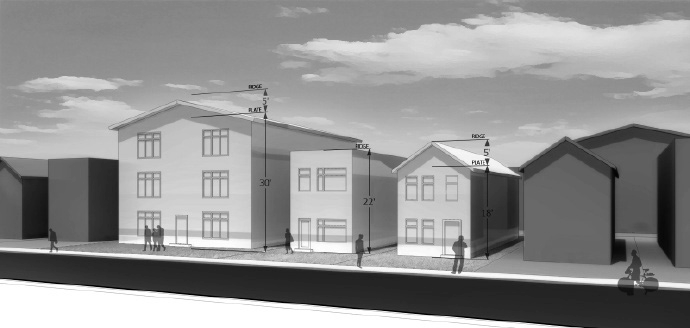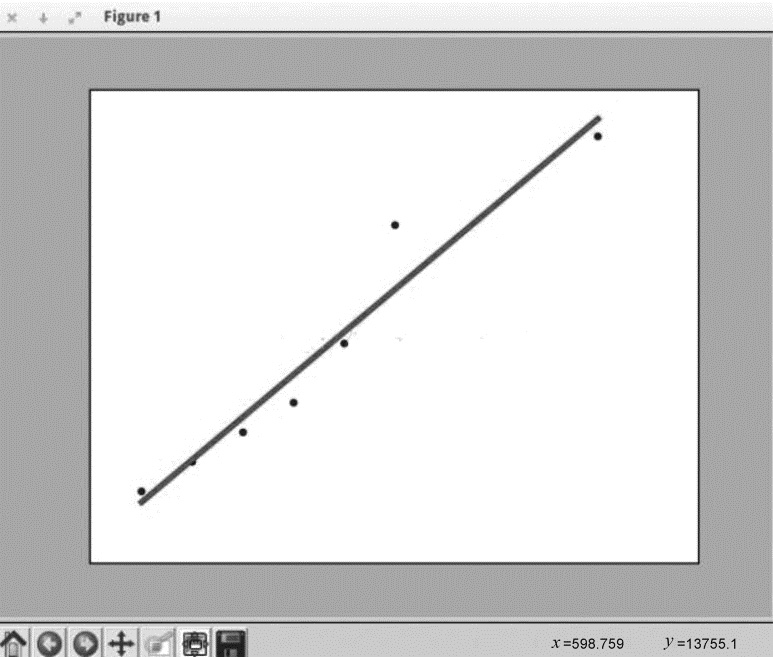# Python 数据挖掘与机器学习实战 (59)：回归分析介 3.3(用 Python 实现一元线性回归)|编号||平方英尺|价格（元 / 平方英尺）|
|-|-|-|
|1|150|6450|
|2|200|7450|
|3|250|8450|
|4|300|9450|
|5|350|11450|
|6|400|15450|
|7|600|18450|

（1）在线性回归中，必须在数据中找出一种线性关系，以使我们可以得到 $a$$b$。假设方程式如下：

$y(X)=a+bX\quad\quad\quad\quad\quad\quad\quad(3-6)$

#需要的包import matplotlib.pyplot as pltimport numpy as npimport pandas as pdfrom sklearn import datasets, linear_model

（2）把数据存储成一个 CSV 文件，命名为 input_data.csv，需要编写一个函数把数据转换为 $X$ 值（平方英尺）、$Y$ 值（价格）。这一步很简单，可以先用 Excel 来存储数据，记得写上列名。之后保存的时候另存为 CSV 格式即可。

01  # 读取数据函数02  def get_data(file_name):03   data = pd.read_csv(file_name)        #读取 cvs 文件04   X_parameter = []05   Y_parameter = []06   for single_square_feet ,single_price_value in zip(data['square_feet'],     data['price']):07         #遍历数据08         X_parameter.append([float(single_square_feet)])                                  #存储在相应的 list 列表中09         Y_parameter.append(float(single_price_value))                                  #存储在相应的 list 列表中10   return X_parameter,Y_parameter

[[150.0], [200.0], [250.0], [300.0], [350.0], [400.0], [600.0]][6450.0, 7450.0, 8450.0, 9450.0, 11450.0, 15450.0, 18450.0]

（3）现在把 X_parameter 和 Y_parameter 拟合为线性回归模型。需要写一个函数，输入为 X_parameters、Y_parameter 和要预测的平方英尺值，返回 a、b 和预测出的价格值。这里使用的是 scikit-learn 机器学习算法包。该算法包是目前 Python 实现的机器算法包中最好用的一个。

01  #将数据拟合到线性模型02  def linear_model_main(X_parameters,Y_parameters,predict_value):03  #创建线性回归对象04   regr = linear_model.LinearRegression()05   regr.fit(X_parameters, Y_parameters)           #训练模型06   predict_outcome = regr.predict(predict_value)07   predictions = {}08   predictions['intercept'] = regr.intercept_09   predictions['coefficient'] = regr.coef_10   predictions['predicted_value'] = predict_outcome11   return predictions

X,Y = get_data('input_data.csv')predictvalue = 700result = linear_model_main(X,Y,predictvalue)print ("Intercept value " , result['intercept'])print ("coefficient" , result['coefficient'])print ("Predicted value: ",result['predicted_value'])

Intercept value 1771.80851064coefficient [ 28.77659574]Predicted value: [ 21915.42553191]

# 显示线性拟合模型的结果def show_linear_line(X_parameters,Y_parameters):#创建线性回归对象 regr = linear_model.LinearRegression() regr.fit(X_parameters, Y_parameters) plt.scatter(X_parameters,Y_parameters,color='blue') plt.plot(X_parameters,regr.predict(X_parameters),color='red',linewidth=4) plt.xticks(()) plt.yticks(()) plt.show()